Anzeige

# Euclids Geometry

16. Jun 2015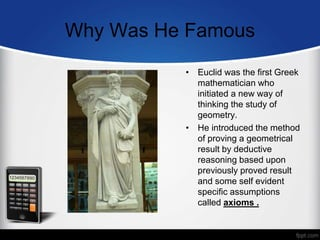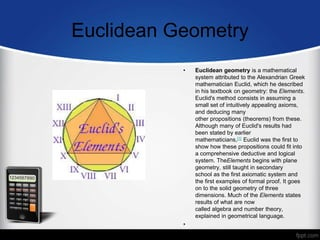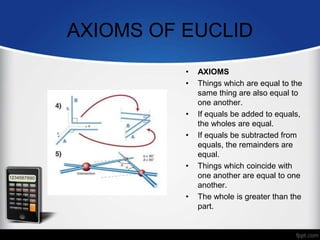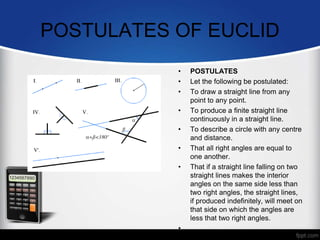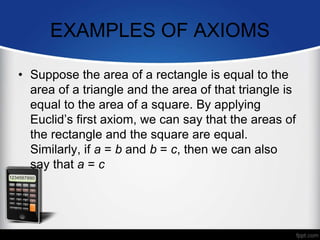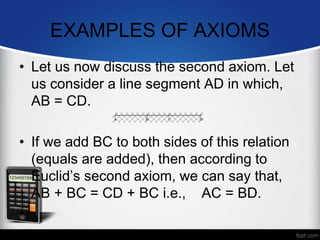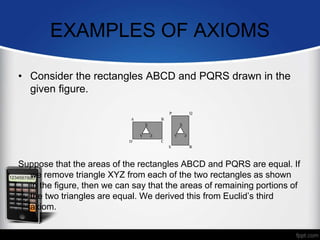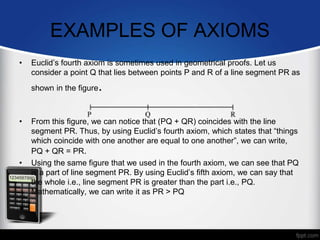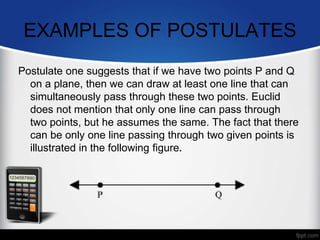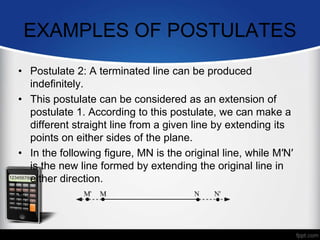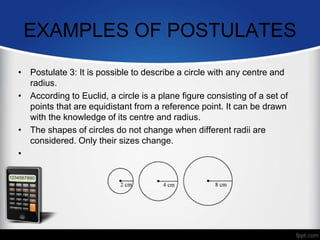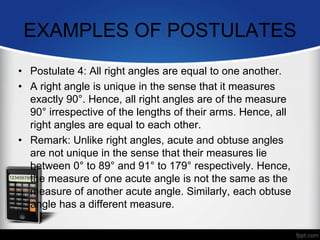1 von 15
Anzeige

### Euclids Geometry

1. EUCLID GEOMETRY MADE BY :- PRATIKSHA MANWATKAR CLASS – IX/M
2. Who Was Euclid Euclid, sometimes called Euclid of Alexandria to distinguish him from Euclid of Megara, was a Greek mathematician, often referred to as the "Father of Geometry". He was active in Alexandria during the reign of Ptolemy I (323–283 BC). His Elements is one of the most influential works in the history of mathematics, serving as the main textbook for teaching mathematics (especially geometr y) from the time of its publication until the late 19th or early 20th century. In the Elements, Euclid deduced the principles of what is now called Euclidean geometry from a small set of axioms. Euclid also wrote works on perspective, conic sections, spherical geometry, number theory and rigor
3. Why Was He Famous • Euclid was the first Greek mathematician who initiated a new way of thinking the study of geometry. • He introduced the method of proving a geometrical result by deductive reasoning based upon previously proved result and some self evident specific assumptions called axioms .
4. Euclidean Geometry • Euclidean geometry is a mathematical system attributed to the Alexandrian Greek mathematician Euclid, which he described in his textbook on geometry: the Elements. Euclid's method consists in assuming a small set of intuitively appealing axioms, and deducing many other propositions (theorems) from these. Although many of Euclid's results had been stated by earlier mathematicians, Euclid was the first to show how these propositions could fit into a comprehensive deductive and logical system. TheElements begins with plane geometry, still taught in secondary school as the first axiomatic system and the first examples of formal proof. It goes on to the solid geometry of three dimensions. Much of the Elements states results of what are now called algebra and number theory, explained in geometrical language. •
5. AXIOMS OF EUCLID • AXIOMS • Things which are equal to the same thing are also equal to one another. • If equals be added to equals, the wholes are equal. • If equals be subtracted from equals, the remainders are equal. • Things which coincide with one another are equal to one another. • The whole is greater than the part.
6. POSTULATES OF EUCLID • POSTULATES • Let the following be postulated: • To draw a straight line from any point to any point. • To produce a finite straight line continuously in a straight line. • To describe a circle with any centre and distance. • That all right angles are equal to one another. • That if a straight line falling on two straight lines makes the interior angles on the same side less than two right angles, the straight lines, if produced indefinitely, will meet on that side on which the angles are less that two right angles. •
7. EXAMPLES OF AXIOMS • Suppose the area of a rectangle is equal to the area of a triangle and the area of that triangle is equal to the area of a square. By applying Euclid’s first axiom, we can say that the areas of the rectangle and the square are equal. Similarly, if a = b and b = c, then we can also say that a = c
8. EXAMPLES OF AXIOMS • Let us now discuss the second axiom. Let us consider a line segment AD in which, AB = CD. • If we add BC to both sides of this relation (equals are added), then according to Euclid’s second axiom, we can say that, AB + BC = CD + BC i.e., AC = BD. •
9. EXAMPLES OF AXIOMS • Consider the rectangles ABCD and PQRS drawn in the given figure. Suppose that the areas of the rectangles ABCD and PQRS are equal. If we remove triangle XYZ from each of the two rectangles as shown in the figure, then we can say that the areas of remaining portions of the two triangles are equal. We derived this from Euclid’s third axiom.
10. EXAMPLES OF AXIOMS • Euclid’s fourth axiom is sometimes used in geometrical proofs. Let us consider a point Q that lies between points P and R of a line segment PR as shown in the figure. • From this figure, we can notice that (PQ + QR) coincides with the line segment PR. Thus, by using Euclid’s fourth axiom, which states that “things which coincide with one another are equal to one another”, we can write, PQ + QR = PR. • Using the same figure that we used in the fourth axiom, we can see that PQ is a part of line segment PR. By using Euclid’s fifth axiom, we can say that the whole i.e., line segment PR is greater than the part i.e., PQ. Mathematically, we can write it as PR > PQ •
11. EXAMPLES OF POSTULATES Postulate one suggests that if we have two points P and Q on a plane, then we can draw at least one line that can simultaneously pass through these two points. Euclid does not mention that only one line can pass through two points, but he assumes the same. The fact that there can be only one line passing through two given points is illustrated in the following figure.
12. EXAMPLES OF POSTULATES • Postulate 2: A terminated line can be produced indefinitely. • This postulate can be considered as an extension of postulate 1. According to this postulate, we can make a different straight line from a given line by extending its points on either sides of the plane. • In the following figure, MN is the original line, while M′N′ is the new line formed by extending the original line in either direction. •
13. EXAMPLES OF POSTULATES • Postulate 3: It is possible to describe a circle with any centre and radius. • According to Euclid, a circle is a plane figure consisting of a set of points that are equidistant from a reference point. It can be drawn with the knowledge of its centre and radius. • The shapes of circles do not change when different radii are considered. Only their sizes change. •
14. EXAMPLES OF POSTULATES • Postulate 4: All right angles are equal to one another. • A right angle is unique in the sense that it measures exactly 90°. Hence, all right angles are of the measure 90° irrespective of the lengths of their arms. Hence, all right angles are equal to each other. • Remark: Unlike right angles, acute and obtuse angles are not unique in the sense that their measures lie between 0° to 89° and 91° to 179° respectively. Hence, the measure of one acute angle is not the same as the measure of another acute angle. Similarly, each obtuse angle has a different measure.
Anzeige